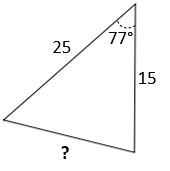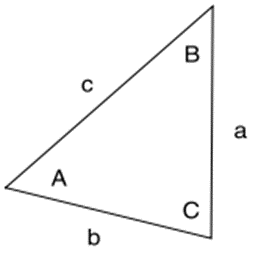## Law of Cosines

For the triangle figure, what is the length of the third side?Hint
Law of Cosines:
$$b^2=a^2+c^2-2ac\:cos\:B$$$Hint 2For oblique (non-right) triangles, use the Law of Cosines to determine unknown sides: $$a^2=b^2+c^2-2bc\:cos\:A$$$
$$b^2=a^2+c^2-2ac\:cos\:B$$$$$c^2=a^2+b^2-2ab\:cos\:C$$$Thus, to find side $$b$$ :
$$b^2=a^2+c^2-2ac\:cos\:B$$$$$b^2=15^2+25^2-2(15)(25)\:cos\:77^{\circ}$$$
$$b^2=225+625-(750)(0.2249)$$$$$b=\sqrt{850-168.71}=\sqrt{681.29}=26.1$$$
26.1